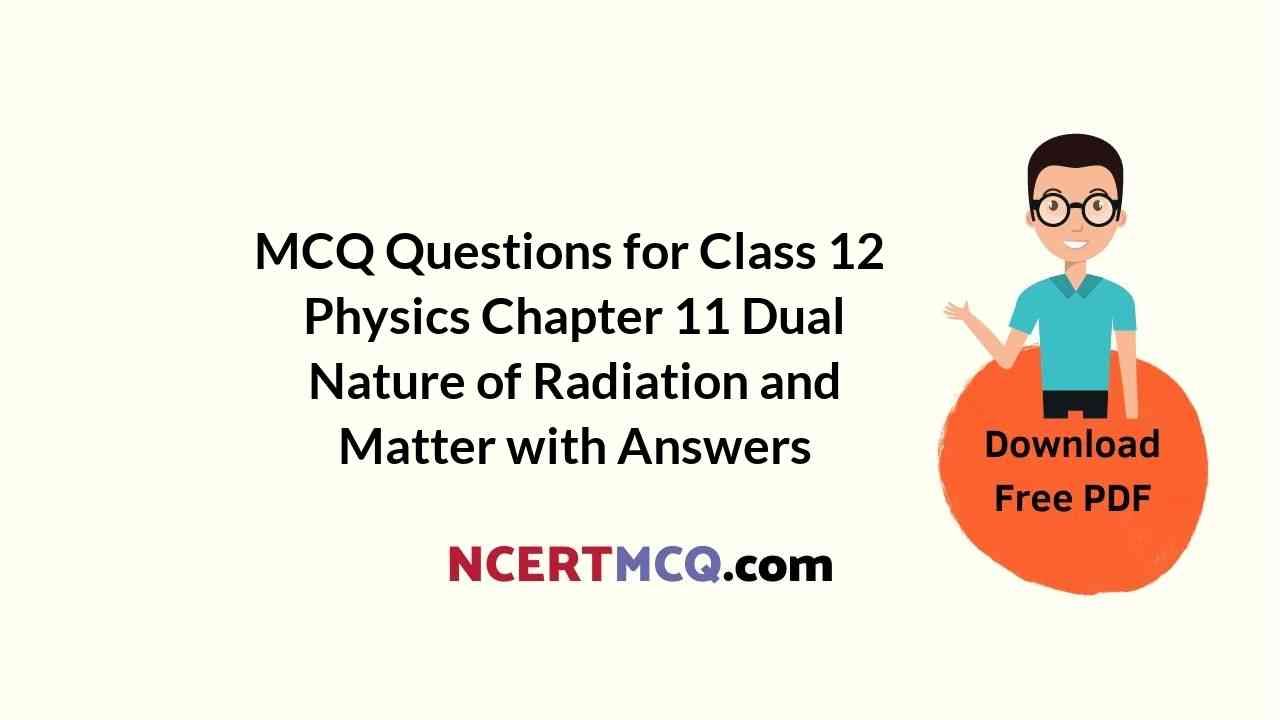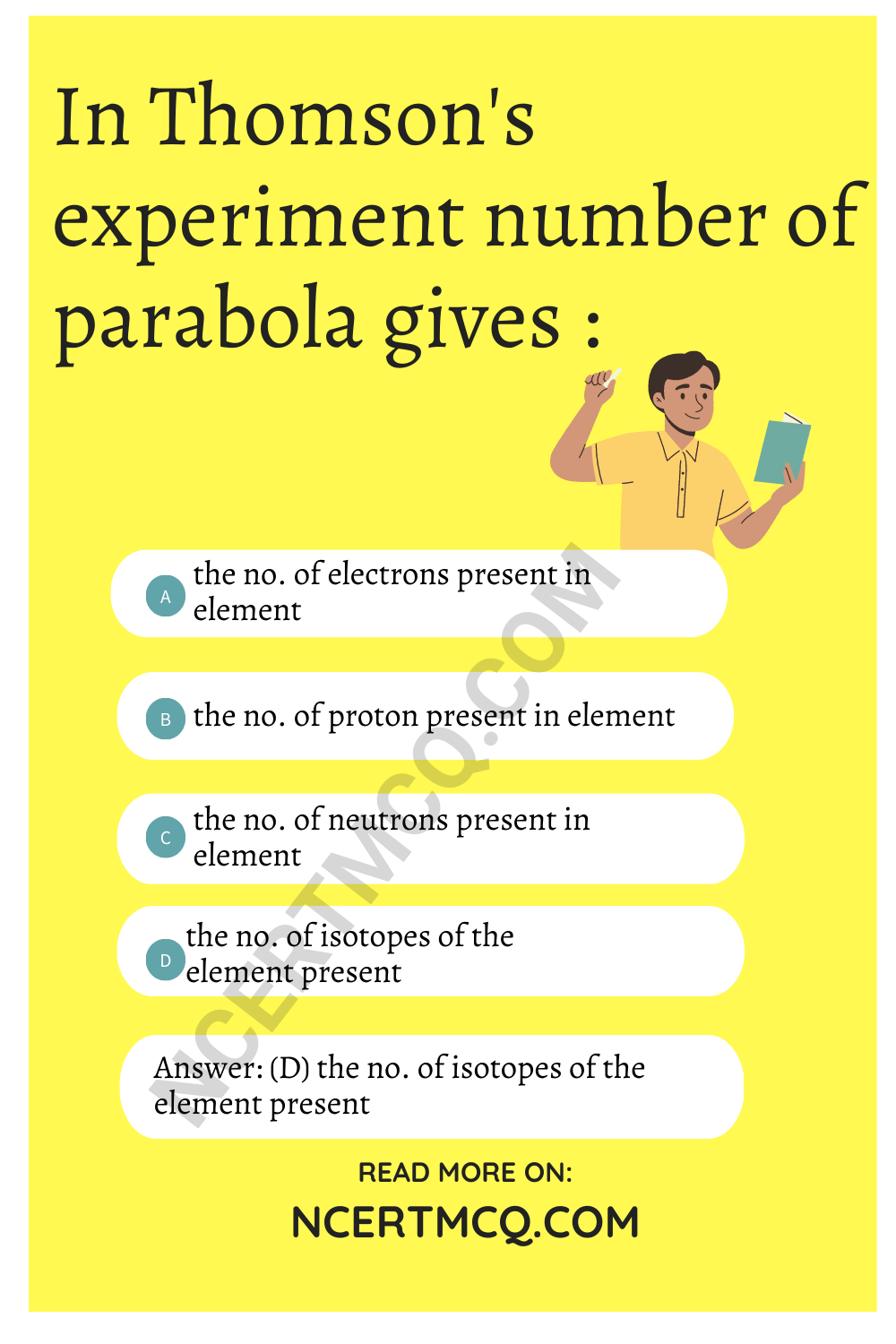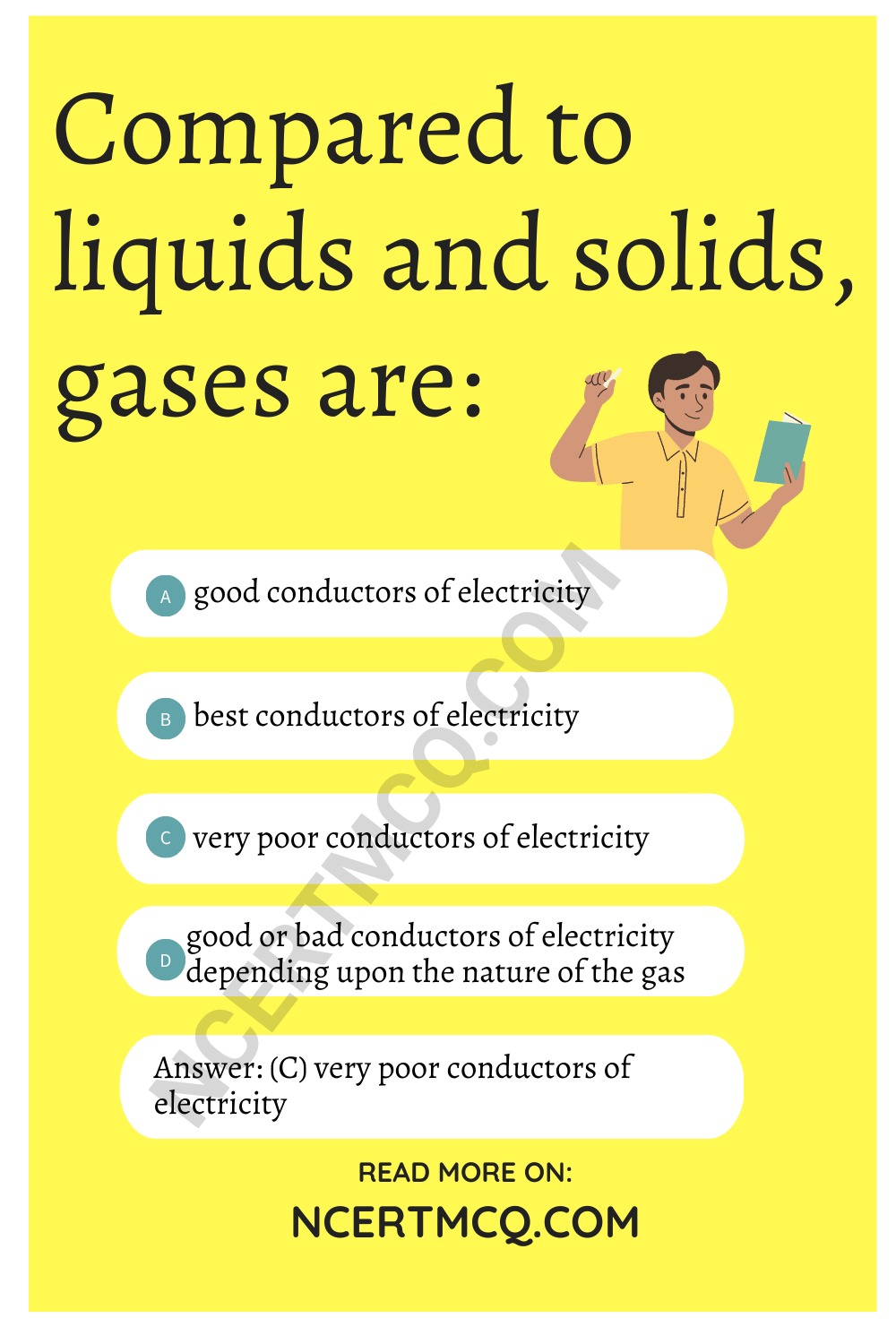Check the below NCERT MCQ Questions for Class 12 Physics Chapter 11 Dual Nature of Radiation and Matter with Answers Pdf free download. MCQ Questions for Class 12 Physics with Answers were prepared based on the latest exam pattern. We have provided Dual Nature of Radiation and Matter Class 12 Physics MCQs Questions with Answers to help students understand the concept very well.

## Dual Nature of Radiation and Matter Class 12 MCQs Questions with Answers

Dual Nature Of Radiation And Matter MCQ Question 1.
De-Broglie equation states the:
(a) dual nature
(b) particle nature
(c) wave nature
(d) none of these

MCQ On Dual Nature Of Matter And Radiation Question 2.
Protons and alpha particles have the same de-Broglie wavelength. What is same for both of them ?
(a) Energy
(b) Time period
(c) Frequency
(d) Momentum

Dual Nature Of Radiation And Matter MCQ Questions With Answers Pdf Question 3.
Kinetic energy of emitted electrons depends upon :
(a) frequency
(b) intensity
(c) nature of atmosphere surrounding the electrons
(d) none of these

De-Broglie wavelength of a body of mass m and kinetic energy E is given by (symbols have their usual meanings):
(a) $$\frac{h}{\sqrt{2mE}}$$
(b) $$\frac{h}{2mE}$$
(c) $$\frac{\sqrt{2mE}}{h}$$
(d) $$\frac{h}{mE}$$

Answer: (a) $$\frac{h}{\sqrt{2mE}}$$

Dual Nature Of Matter And Radiation Class 12 MCQ Question 5.
The ratio of specific charge of an alpha particle to the proton is:
(a) 1 : 2
(b) 2 : 1
(c) 4 : 1
(d) 1 : 4

MCQs On Dual Nature Of Radiation And Matter Question 6.
In Thomson’s experiment number of parabola gives :
(a) the no. of electrons present in element
(b) the no. of proton present in element
(c) the no. of neutrons present in element
(d) the no. of isotopes of the element present

Answer: (d) the no. of isotopes of the element presentMCQ Of Dual Nature Of Radiation And Matter Question 7.
The work function of photoelectric material is 3.3 eV. The threshold frequency will be equal to:
(a) 8 × 1014 Hz
(b) 8 × 1010 Hz
(c) 5 × 1010 Hz
(d) 4 × 1014 Hz

Answer: (a) 8 × 1014 Hz

Question 8.
The strength of photoelectric current depends upon :
(d) distance between anode and cathode

Question 9.
The momentum of an electron that emits a wavelength of 2 Å. will be:
(a) 6.4 × 10-36 kgms-1
(b) 3.3 × 10-24 kgms-1
(c) 3.3 × 10-34 kgms-1
(d) none of these

Answer: (b) 3.3 × 10-24 kgms-1

Question 10.
Name the scientists who first studied the passage of electricity through fluids to establish the electrical nature of matter:
(a)Millikan
(b) Planck
(d) Boyle

Question 11.
Millikan’s oil drop experiment makes use of:
(a) Stokes’ law
(b) Boyle’s law
(c) Gas equation
(d) Bernoulli’s theorem

Question 12.
X-rays are:
(a) deflected by an electric field
(b) deflected by a magnetic field
(c) deflected by both electric and magnetic fields
(d) not deflected by electric and magnetic fields

Answer: (d) not deflected by electric and magnetic fields

Question 13.
In photo electric emission, for alkali metals the threshold frequency lies in the:
(a) visible region
(b) ultraviolet region
(c) infrared region
(d) far end of the infrared region

Question 14.
Which of the following radiations cannot eject photo electrons?
(a) ultraviolet
(b) infrared
(c) visible
(d) X-rays

Question 15.
What is the de-Broglie wavelength of an electron accelerated from rest through a potential difference of V volts?
(a) $$\frac{12.3}{V^2}$$ Å
(b) $$\frac{12.3}{V}$$ Å
(c) $$\frac{12.2}{V^2}$$ Å
(d) None of these

Answer: (a) $$\frac{12.3}{V^2}$$ Å

Question 16.
What is the de-Broglie wavelength of an electron accelerated from rest through a potential difference of 100 volts?
(a) 12.3 Å
(b) 1.23 Å
(c) 0.123 Å
(d) None of these

Question 17.
What is the de-Broglie wavelength of a proton accelerated from rest through a potential difference of V volts?
(a) $$\frac{12.3}{√V}$$ Å
(b) $$\frac{12.3}{V}$$ Å
(c) $$\frac{12.2}{V^2}$$ Å
(d) None of these

Question 18.
When a yellow light is incident on a surface, no electrons are emitted while green light can emit electrons. If the red light is incident on the surface then:
(a) no electrons are emitted
(b) photons are emitted
(c) electrons of higher energy are emitted
(d) electrons of lower energy are emitted

Answer: (a) no electrons are emitted

Question 19.
The de-Broglie wavelength of particle of mass 1 mg moving with a velocity of 1 ms-1, in terms of Planck’s constant h, is given by (in metre):
(a) 105 h
(b) 106 h
(c) 10-3 h
(d) 103 h

Question 20.
Evidence of the wave nature of light cannot be obtained from:
(a) diffraction
(b) interference
(c) doppler effect
(d) reflection

Question 21.
which Characteristic of a target does the Mosley’s law relate the frequency of X-rays?
(a) density
(b) atomic number
(c) atomic weight
(d) interatomic space

Question 22.
The charge of a photo electron is :
(a) 9.1 × 10-31 C
(b) 9.1 × 10-27 C
(c) 9.1 × 10-24 C
(d) none of these

Question 23.
The number of photons of frequency n present in energy E, in terms of Planck’s constant h:
(a) $$\frac{E}{nh}$$
(b) nhE
(c) $$\frac{nh}{E}$$
(d) $$\frac{nE}{h}$$

Answer: (a) $$\frac{E}{nh}$$

Question 24.
Compared to liquids and solids, gases are:
(a) good conductors of electricity
(b) best conductors of electricity
(c) very poor conductors of electricity
(d) good or bad conductors of electricity depending upon the nature of the gas

Answer: (c) very poor conductors of electricityQuestion 25.
The different stages of discharge in a discharge tube can be explained on the basis of:
(a) the wave nature of light
(b) the dual nature of light
(c) wave nature of electrons
(d) the collision between the charged particles emitted from the cathode the atoms of the gas in the tube

Answer: (d) the collision between the charged particles emitted from the cathode the atoms of the gas in the tube

Question 26.
When an electron jumps across a potential difference of 1 V, it gains energy equal to :
(a) 1.602 × 10-19 J
(b) 1.602 × 1019 J
(c) 1.602 × 1024 J
(d) 1 J